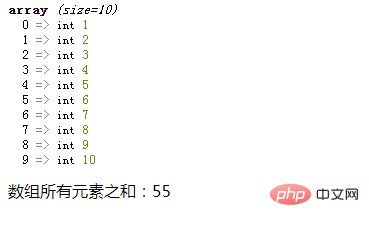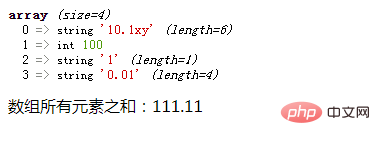# PHP数组学习之计算数组元素总和

```<?php
\$array= array(1,2,3,4,5,6,7,8,9,10);
\$sum=0;
for (\$i=0; \$i < count(\$array); \$i++) {
\$sum+=\$array[\$i];
}
echo '1 + 2 + 3 +...+ 9 + 10 = '. \$sum;
?>```

`1 + 2 + 3 +...+ 9 + 10 = 55`

```<?php
\$array= array(1,2,3,4,5,6,7,8,9,10);
\$sum=0;
foreach (\$array as \$value) {
\$sum+=\$value;
}
echo '数组所有元素之和：'. \$sum;
?>```

`数组所有元素之和：55`

foreach循环语句中，遍历给定的 `\$array` 数组，在每次循环中将当前数组的值赋给 `\$value`

array_sum()是PHP中的一个内置函数，可以计算数组中所有元素的和，并返回元素之和。

```<?php
\$array= array(1,2,3,4,5,6,7,8,9,10);
var_dump(\$array);
echo '数组所有元素之和：'. array_sum(\$array);
?>```• 如果`\$array`中所有元素都是整数，则返回一个整数值；如果其中有一个或多个值是浮点数，则返回浮点数。

• 如果`\$array`中存在非数值类型的元素，那么PHP会将它们转换成一个数值（PHP是弱语言类型，会根据变量的值，自动把变量转换为正确的数据类型），转换失败就作为 `0` 值来参加计算。

```<?php
\$array= array("10.1xy", 100, '1', "0.01");
var_dump(\$array);
echo '数组所有元素之和：'. array_sum(\$array);
?>```### 全部评论我要评论

• 取消发布评论发送
• 1/1